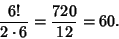## Pascal's TheoremThe dual of Brianchon's Theorem. It states that, given a (not necessarily Regular, or even Convex) Hexagon inscribed in a Conic Section, the three pairs of the continuations of opposite sides meet on a straight Line, called the Pascal Line. There are 6! (6! means 6 Factorial, where) possible ways of taking all Vertices in any order, but among these are six equivalent Cyclic Permutations and two possible orderings, so the total number of different hexagons (not all simple) isThere are therefore a total of 60 Pascal Lines created by connecting Vertices in any order. These intersect three by three in 20 Steiner Points.

See also Braikenridge-Maclaurin Construction, Brianchon's Theorem, Cayley-Bacharach Theorem, Conic Section, Duality Principle, Hexagon, Pappus's Hexagon Theorem, Pascal Line, Steiner Points

References

Coxeter, H. S. M. and Greitzer, S. L. Geometry Revisited. Washington, DC: Math. Assoc. Amer., pp. 73-76, 1967.

Ogilvy, C. S. Excursions in Geometry. New York: Dover, pp. 105-106, 1990.

Pappas, T. The Mystic Hexagram.'' The Joy of Mathematics. San Carlos, CA: Wide World Publ./Tetra, p. 118, 1989.